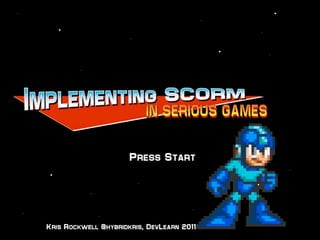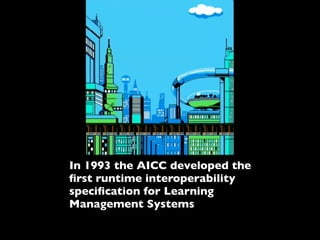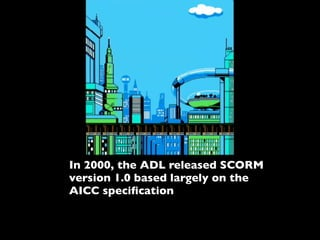Successfully reported this slideshow.

# Implementing SCORM in Serious Games×

# Implementing SCORM in Serious Games

The presentation was given at DevLearn 2011 and covers SCORM in games, metrics in games, a case study and a look at how the new CMI5 spec MAY be useful for tracking learning data in games.

NOTE- Slideshare didn't like all of my transitions or the video I included in the original presentation. I have put a URL in place of the video and you'll just have to use your imagination for the transitions. It's a shame too because the transitions really made the presentation. If you want the full presentation with the video and transitions please contact me on twitter @hybridkris.

The presentation was given at DevLearn 2011 and covers SCORM in games, metrics in games, a case study and a look at how the new CMI5 spec MAY be useful for tracking learning data in games.

NOTE- Slideshare didn't like all of my transitions or the video I included in the original presentation. I have put a URL in place of the video and you'll just have to use your imagination for the transitions. It's a shame too because the transitions really made the presentation. If you want the full presentation with the video and transitions please contact me on twitter @hybridkris.

### Implementing SCORM in Serious Games

1. 1. In 1993 the AICC developed the ﬁrst runtime interoperability speciﬁcation for Learning Management Systems
2. 2. In 2000, the ADL released SCORM version 1.0 based largely on the AICC speciﬁcation
3. 3. Since the implementation, both SCORM and the CMI specs have allowed for excellent courseware tracking capabilities
4. 4. Courseware, however, has changed over the past 18 years...
5. 5. How can we adapt the SCORM model to interface with new courseware models such as gaming?...
6. 6. Games are engaging and becoming more prominent as a learning tool (and they’re fun!).
7. 7. Games can be cost effective and easy to implement in curriculum
8. 8. Games provide a wide range of interactions and can be customized to users
9. 9. Captured game data can provide rich, deep metrics for analysis
10. 10. Level Clear!!
11. 11. Game Metrics Examples
12. 12. Call of Duty: Black Ops Metrics http://www.codblackopsblog.com/wp-content/uploads/2011/01/the_numbers_mason_V2.jpg
13. 13. Call of Duty: Black Ops Metrics ti sa T ha T LO a! of dat http://www.codblackopsblog.com/wp-content/uploads/2011/01/the_numbers_mason_V2.jpg
14. 14. Starcraft/Starcraft II Metrics http://us.blizzard.com/_images/games/sc2/wallpapers/wall1/wall1-1600x1200.jpg http://images.wikia.com/starcraft/images/c/cb/Screenshot_SC2_DevGame1.jpg
15. 15. Starcraft/Starcraft II Metrics http://us.blizzard.com/_images/games/sc2/wallpapers/wall1/wall1-1600x1200.jpg http://images.wikia.com/starcraft/images/c/cb/Screenshot_SC2_DevGame1.jpg
16. 16. http://arstechnica.com/gaming/news/2010/07/excellence-of- execution-video-of-starcraft-mastery.ars (SlideShare didn’t like my video)
17. 17. That’s 300 actions PER MINUTE http://arstechnica.com/gaming/news/2010/07/excellence-of- execution-video-of-starcraft-mastery.ars (SlideShare didn’t like my video)
18. 18. http://arstechnica.com/gaming/news/2010/07/excellence-of-execution-video-of-starcraft-mastery.ars
19. 19. Civilization V http://arstechnica.com/gaming/news/2010/07/excellence-of-execution-video-of-starcraft-mastery.ars
20. 20. Civilization V Operation FlashPoint http://arstechnica.com/gaming/news/2010/07/excellence-of-execution-video-of-starcraft-mastery.ars
21. 21. Case Study: “The Change Game”
22. 22. Simple game utilizing decision tree and quiz components
23. 23. Web based game developed in Flash Simple game utilizing decision tree and quiz components
24. 24. Very simple mechanics that were to be tracked Web based game developed in Flash Simple game utilizing decision tree and quiz components
25. 25. Very simple mechanics that were to be tracked Web based game developed in Flash Simple game utilizing decision tree and quiz components
26. 26. Multiple choice style questions cmi.core.score.raw
27. 27. Free form journal entries and self assessment forms cmi.suspend.data Multiple choice style questions cmi.core.score.raw
28. 28. Completion of game cmi.suspend.data cmi.core.lesson_status Overall time in game cmi.core.total_time Free form journal entries and self assessment forms cmi.suspend.data Multiple choice style questions cmi.core.score.raw
29. 29. Completion of game cmi.suspend.data cmi.core.lesson_status Overall time in game cmi.core.total_time Free form journal entries and self assessment forms cmi.suspend.data Multiple choice style questions cmi.core.score.raw
30. 30. Results
31. 31. Results What went right
32. 32. Results What went right - Data was tracked and reported to the LMS
33. 33. Results What went right - Data was tracked and reported to the LMS - Implementation was easy using standard SCORM calls
34. 34. Results What went right - Data was tracked and reported to the LMS - Implementation was easy using standard SCORM calls - SCORM data model elements were easily adapted to the required functionality
35. 35. Results
36. 36. Results What could be improved
37. 37. Results What could be improved - Journal entries are not truly “tracked”
38. 38. Results What could be improved - Journal entries are not truly “tracked” - Game play is limited and doesn’t really reﬂect more common game play types
39. 39. Results What could be improved - Journal entries are not truly “tracked” - Game play is limited and doesn’t really reﬂect more common game play types - If the game was “off the shelf”, SCORM integration would have been far more difﬁcult
40. 40. Results What could be improved - Journal entries are not truly “tracked” - Game play is limited and doesn’t really reﬂect more common game play types - If the game was “off the shelf”, SCORM integration would have been far more difﬁcult - No tracking implemented to track the user through the entire experience
41. 41. Activating CMI 5!!!
42. 42. CMI-5 is the next generation of Content-to-LMS interoperability speciﬁcations. It will provide content runtime data tracking and a means to transfer course lists.
43. 43. CMI-5 is intended to replace existing AICC and SCORM speciﬁcations
44. 44. “Core” set of minimum pre-deﬁned data elements for content tracking
45. 45. Ability for the content to deﬁne what data to be collected
46. 46. Game Over
47. 47. Kris Rockwell, CEO Hybrid Learning Systems @hybridkris kris.rockwell@hybrid-learning.com www.hybrid-learning.com

• \n
• \n
• \n
• \n
• \n
• \n
• \n
• \n
• \n
• \n
• \n
• \n
• \n
• \n
• \n
• \n
• \n
• \n
• \n
• \n
• \n
• \n
• \n
• \n
• \n
• \n
• \n
• \n
• \n
• \n
• \n
• \n
• \n
• \n
• \n
• \n
• \n
• \n
• \n
• \n
• \n
• \n
• \n
• \n
• \n
• \n
• \n
• \n
• \n
• \n
• \n
• \n
• \n
• \n
• \n
• \n
• \n
• \n
• \n
• \n
• \n
• \n
• \n
• \n
• \n
• \n
• \n
• \n
• \n
• \n
• \n
• \n
• \n
• \n
• \n
• \n
• \n
• \n
• \n
• \n
• \n
• \n
• \n
• \n
• \n
• \n
• \n
• \n
• \n
• \n
• \n
• \n
• \n
• \n
• \n
• \n
• \n
• \n
• \n
• \n
• \n
• \n
• \n
• \n
• \n
• \n
• \n
• \n
• \n
• \n
• \n
• \n
• \n
• \n
• \n
• \n
• \n
• \n
• \n
• \n
• \n
• \n
• \n
• \n
• \n
• \n
• \n
• \n
• \n
• \n
• \n
• \n
• \n
• \n
• \n
• \n
• \n
• \n
• \n
• \n
• \n
• \n
• \n
• \n
• \n
• \n
• \n
• \n
• \n
• \n
• \n
• \n
• \n
• \n
• \n
• \n
• \n
• \n
• \n
• \n
• \n
• \n
• \n
• \n
• \n
• \n
• \n
• \n
• \n
• \n
• \n
• \n
• \n
• \n
• \n
• \n
• \n
• \n
• \n
• \n
• \n
• \n
• \n
• \n
• \n
• \n
• \n
• \n
• \n
• \n
• \n
• \n
• \n
• \n
• \n
• \n
• \n
• \n
• \n
• \n
• \n
• \n
• \n
• \n
• \n
• \n
• \n
• \n
• \n
• \n
• \n
• \n
• \n
• \n
• \n
• \n
• \n
• \n
• \n
• \n
• \n
• \n
• \n
• \n
• \n
• \n
• \n
• \n
• \n
• \n
• \n
• \n
• \n
• \n
• \n
• \n
• \n
• \n
• \n
• \n
• \n
• \n
• \n
• \n
• \n
• \n
• \n
• \n
• \n
• \n
• \n
• \n
• \n
• \n
• \n
• \n
• \n
• \n
• \n
• \n
• \n
• \n
• \n
• \n
• \n
• \n
• \n
• \n
• \n
• \n
• \n
• \n
• \n
• \n
• \n
• \n
• \n
• \n
• \n
• \n
• \n
• \n
• \n
• \n
• \n
• \n
• \n
• \n
• \n
• \n
• \n
• \n
• \n
• \n
• \n
• \n
• \n
• \n
• \n
• \n
• \n
• \n
• \n
• \n
• \n
• \n
• \n
• \n
• \n
• \n
• \n
• \n
• \n
• \n
• \n
• \n
• \n
• \n
• \n
• \n
• \n
• \n
• \n
• \n
• \n
• \n
• \n
• \n
• \n
• \n
• \n
• \n
• \n
• \n
• \n
• \n
• \n
• \n
• \n
• \n
• \n
• \n
• \n
• \n
• \n
• \n
• \n
• \n
• \n
• \n
• \n
• \n
• \n
• \n
• \n
• \n
• \n
• \n
• \n
• \n
• \n
• \n
• \n
• \n
• \n
• \n
• \n
• \n
• \n
• \n
• \n
• \n
• \n
• \n
• \n
• \n
• \n
• \n
• \n
• \n
• \n
• \n
• \n
• \n
• \n
• \n
• \n
• \n
• \n
• \n
• \n
• \n
• \n
• \n
• \n
• \n
• \n
• \n
• \n
• \n
• \n
• \n
• \n
• \n
• \n
• \n
• \n
• \n
• \n
• \n
• \n
• \n
• \n
• \n
• \n
• \n
• \n
• \n
• \n
• \n
• \n
• \n
• \n
• \n
• \n
• \n
• \n
• \n
• \n
• \n
• \n
• \n
• \n
• \n
• \n
• \n
• \n
• \n
• \n
• \n
• \n
• \n
• \n
• \n
• \n
• \n
• \n
• \n
• \n
• \n
• \n
• \n
• \n
• \n
• \n
• \n
• \n
• \n
• \n
• \n
• \n
• \n
• \n
• \n
• \n
• \n
• \n
• \n
• \n
• \n
• \n
• \n
• \n
• \n
• \n
• \n
• \n
• \n
• \n
• \n
• \n
• \n
• \n
• \n
• \n
• \n
• \n
• \n
• \n
• \n
• \n
• \n
• \n
• \n
• \n
• \n
• \n
• \n
• \n
• \n
• \n
• \n
• \n
• \n
• \n
• \n
• \n
• \n
• \n
• \n
• \n
• \n
• \n
• \n
• \n
• \n
• \n
• \n
• \n
• \n
• \n
• \n
• \n
• \n
• \n
• \n
• \n
• \n
• \n
• \n
• \n
• \n
• \n
• \n
• \n
• \n
• \n
• \n
• \n
• \n
• \n
• \n
• \n
• \n
• \n
• \n
• \n
• \n
• \n
• \n
• \n
• \n
• \n
• \n
• \n
• \n
• \n
• \n
• \n
• \n
• \n
• \n
• \n
• \n
• \n
• \n
• \n
• \n
• \n
• \n
• \n
• \n
• \n
• \n
• \n
• \n
• \n
• \n
• \n
• \n
• \n
• \n
• \n
• \n
• \n
• \n
• \n
• \n
• \n
• \n
• \n
• \n
• \n
• \n
• \n
• \n
• \n
• \n
• \n
• \n
• \n
• \n
• \n
• \n
• \n
• \n
• \n
• \n
• \n
• \n
• \n
• \n
• \n
• \n
• \n
• \n
• \n
• \n
• \n
• \n
• \n
• \n
• \n
• \n
• \n
• \n
• \n
• \n
• \n
• \n
• \n
• \n
• \n
• \n
• \n
• \n
• \n
• \n
• \n
• \n
• \n
• \n
• \n
• \n
• \n
• \n
• \n
• \n
• \n
• \n
• \n
• \n
• \n
• \n
• \n
• \n
• \n
• \n
• \n
• \n
• \n
• \n
• \n
• \n
• \n
• \n
• \n
• \n
• \n
• \n
• \n
• \n
• \n
• \n
• \n
• \n
• \n
• \n
• \n
• \n
• \n
• \n
• \n
• \n
• \n
• \n
• \n
• \n
• \n
• \n
• \n
• \n